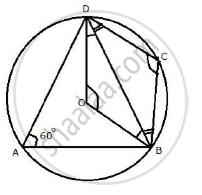Share

Abcd is a Quadrilateral Inscribed in a Circle, Having ∠ = 60°; O is the Center of the Circle. Show That: ∠Obd + ∠Odb =∠Cbd +∠Cdb - ICSE Class 10 - Mathematics

ConceptArc and Chord Properties - the Angle that an Arc of a Circle Subtends at the Center is Double that Which It Subtends at Any Point on the Remaining Part of the Circle

Question

ABCD is a quadrilateral inscribed in a circle, having ∠ = 60°; O is the center of the circle. Show that:
∠OBD + ∠ODB
=∠CBD +∠CDB

Solution∠ BOD =  2 ∠BAD =  2 × 60°  = 120°
And  ∠ BCD  = 1/2 Refelx  (∠BOD) ( 360° - 120°) =  120°
(Angle at the centre is double the angle at the circumference subtended by the same chord
∴  ∠CBD + ∠CDB =180° - 120° = 60°
(By angle sum property of triangle CBD)
Again, ∠OBD+ ∠ODB=180° - 120° = 60°
(By angle sum property of triangle OBD)
∴ ∠OBD + ∠ODB = ∠CBD+ ∠CDB

Is there an error in this question or solution?

Video TutorialsVIEW ALL 

Solution Abcd is a Quadrilateral Inscribed in a Circle, Having ∠ = 60°; O is the Center of the Circle. Show That: ∠Obd + ∠Odb =∠Cbd +∠Cdb Concept: Arc and Chord Properties - the Angle that an Arc of a Circle Subtends at the Center is Double that Which It Subtends at Any Point on the Remaining Part of the Circle.
S